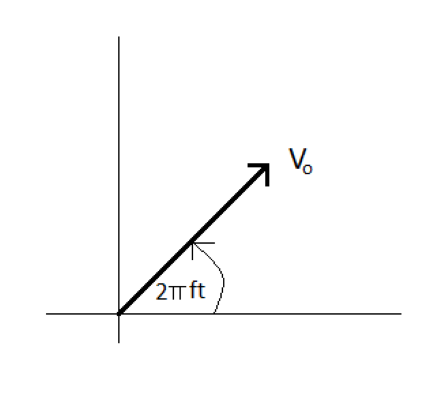# The maximum voltage V_o supplied by the generator in an ac circuit can be represented by a...

## Question:

The maximum voltage {eq}V_o {/eq} supplied by the generator in an ac circuit can be represented by a rotating phasor (see the drawing). The value of the instantaneous voltage {eq}V {/eq} at a time {eq}t {/eq} is given by {eq}\text{_______} {/eq}(a) the angle between the phasor and the horizontal axis.

(b) the component of the phasor along the vertical axis.

(c) the component of the phasor along the horizontal axis.

(d) the length of the phasor.

## Phasor Diagram

Alternating current or AC is a sinusoidally varying one. The voltage across an AC circuit can be represented by a periodic function of the form {eq}V = V_0 \sin ( \omega t + \phi ) {/eq}. Here {eq}V_0, \ \ \omega, \ \ \phi {/eq} are the voltage amplitude, angular frequency of the voltage, and phase constant. The phasor diagram is used for representing the instantaneous AC voltage or current against the reference axis, the x axis. The angle between the phasor and the reference axis gives the phase lead or lag of the quantity represented by the phasor.

• The length of the phasor represents the magnitude of the quantity represented by the phasor.
• The direction of the phasor indicates the phase lag or lead of the represented quantity.

Given data

• Maximum voltage {eq}V_0 {/eq}
• The rotating phasor represents the voltage supplied by the generator.

Part a )

The angle made by the phasor with the reference axis, the x axis at the given instant {eq}\theta = 2\pi F t {/eq}

Part b )

The component of the phasor along the vertical axis {eq}V_y = V_0 \sin ( 2 \pi F t ) {/eq}

Part c )

The component of phasor along the horizontal axis {eq}V _x = V_0 \cos ( 2 \pi F t ) {/eq}

Part d )

Length of the phasor {eq}V_m = \sqrt { V_0^2 \sin^2 ( 2 \pi F t ) + V_0^2 \cos^2 ( 2 \pi F t ) } \\ V_m = V_0 {/eq}